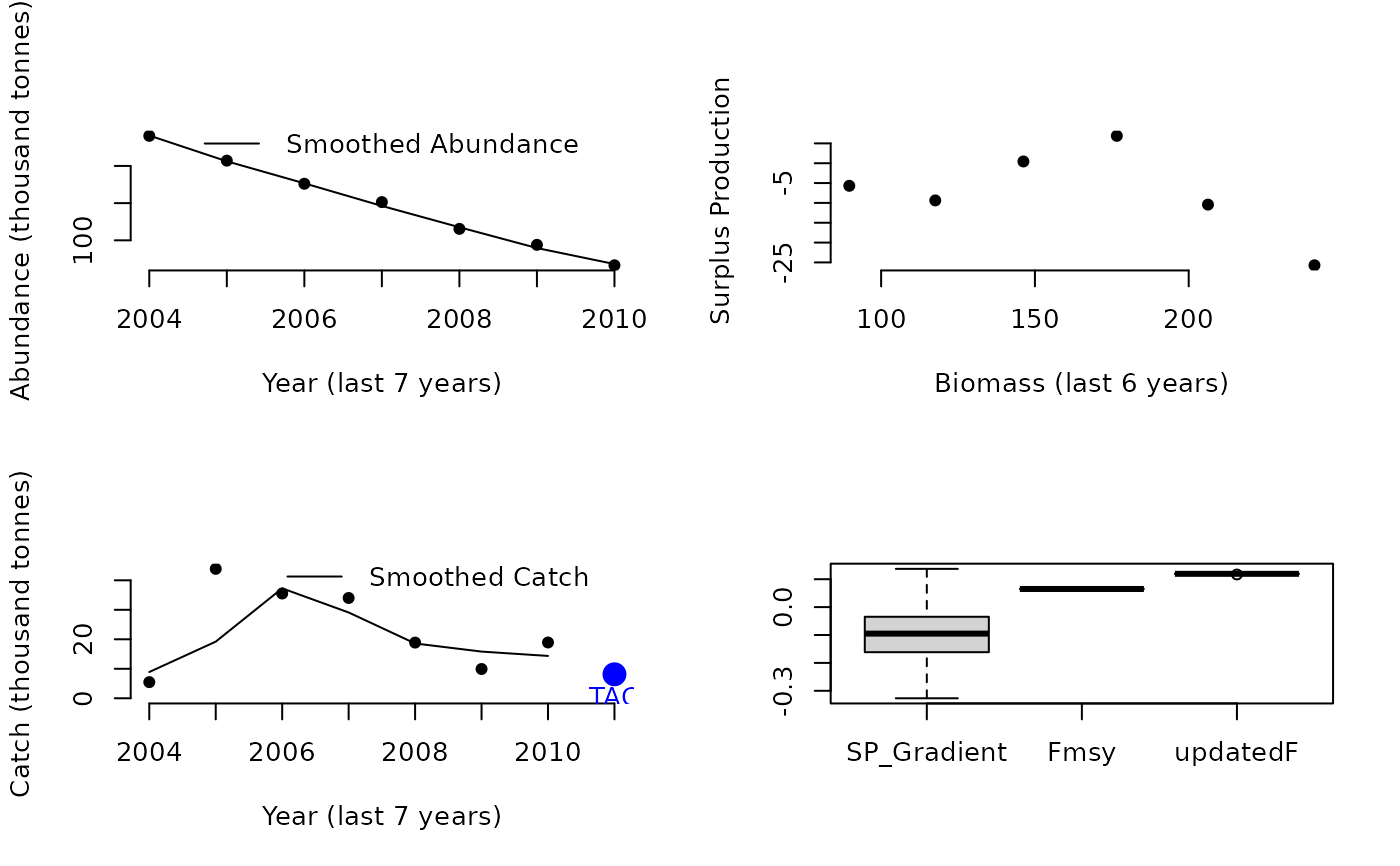An adaptive MP that uses trajectory in inferred suplus production and fishing mortality rate to update a TAC

Fadapt(x, Data, reps = 100, plot = FALSE, yrsmth = 7, gg = 1)

## Arguments

x

A position in the data object

Data

A data object

reps

The number of stochastic samples of the MP recommendation(s)

plot

Logical. Show the plot?

yrsmth

Years over which to smooth recent estimates of surplus production

gg

A gain parameter controlling the speed in update in TAC.

## Value

An object of class Rec-class with the TAC slot populated with a numeric vector of length reps

A numeric vector of quota recommendations

## Details

Fishing rate is modified each year according to the gradient of surplus production with biomass (aims for zero). F is bounded by FMSY/2 and 2FMSY and walks in the logit space according to dSP/dB. This is derived from the theory of Maunder 2014.

The TAC is calculated as: $$\textrm{TAC}_y= F_y B_{y-1}$$ where $$B_{y-1}$$ is the most recent biomass, estimated with a loess smoother of the most recent yrsmth years from the index of abundance (Data@Ind) and estimate of current abundance (Data@Abun), and

$$F_y = F_{\textrm{lim}_1} + \left(\frac{\exp^{F_{\textrm{mod}_2}}} {1 + \exp^{F_{\textrm{mod}_2}}} F_{\textrm{lim}_3} \right)$$

where $$F_{\textrm{lim}_1} = 0.5 \frac{F_\textrm{MSY}}{M}M$$, $$F_{\textrm{lim}_2} = 2 \frac{F_\textrm{MSY}}{M}M$$, $$F_{\textrm{lim}_3}$$ is $$F_{\textrm{lim}_2} - F_{\textrm{lim}_1}$$, $$F_{\textrm{mod}_2}$$ is $$F_{\textrm{mod}_1} + g -G$$ where $$g$$ is gain parameter gg, G is the predicted surplus production given current abundance, and: $$F_{\textrm{mod}_1} = \left\{\begin{array}{ll} -2 & \textrm{if } F_\textrm{old} < F_{\textrm{lim}_1} \\ 2 & \textrm{if } F_\textrm{old} > F_{\textrm{lim}_2} \\ \log{\frac{F_\textrm{frac}}{1-F_\textrm{frac}}} & \textrm{if } F_{\textrm{lim}_1} \leq F_\textrm{old} \leq F_{\textrm{lim}_2} \\ \end{array}\right.$$ where $$-F_{\textrm{frac}} = \frac{F_{\textrm{old}} - F_{\textrm{lim}_1}}{F_{\textrm{lim}_3}}$$, $$F_\textrm{old} = \sum{\frac{C_\textrm{hist}}{B_\textrm{hist}}}/n$$ where $$C_\textrm{hist}$$ and $$B_\textrm{hist}$$ are smooth catch and biomass over last yrsmth, and $$n$$ is yrsmth.

Tested in Carruthers et al. 2015.

## Required Data

See Data-class for information on the Data object

Fadapt: Abun, Cat, FMSY_M, Ind, Mort, Year

## Rendered Equations

See Online Documentation for correctly rendered equations

Other Fmsy/M methods: DynF(), Fratio()

Other Surplus production MPs: Rcontrol(), SPMSY(), SPSRA(), SPmod(), SPslope()

T. Carruthers

## Examples

Fadapt(1, Data=MSEtool::Atlantic_mackerel, plot=TRUE)#> TAC (median)
#>     8.126264